Alternating Current NAT Level - 2

# Alternating Current NAT Level - 2

Test Description

## 10 Questions MCQ Test Topic wise Tests for IIT JAM Physics | Alternating Current NAT Level - 2

Alternating Current NAT Level - 2 for Physics 2022 is part of Topic wise Tests for IIT JAM Physics preparation. The Alternating Current NAT Level - 2 questions and answers have been prepared according to the Physics exam syllabus.The Alternating Current NAT Level - 2 MCQs are made for Physics 2022 Exam. Find important definitions, questions, notes, meanings, examples, exercises, MCQs and online tests for Alternating Current NAT Level - 2 below.
Solutions of Alternating Current NAT Level - 2 questions in English are available as part of our Topic wise Tests for IIT JAM Physics for Physics & Alternating Current NAT Level - 2 solutions in Hindi for Topic wise Tests for IIT JAM Physics course. Download more important topics, notes, lectures and mock test series for Physics Exam by signing up for free. Attempt Alternating Current NAT Level - 2 | 10 questions in 45 minutes | Mock test for Physics preparation | Free important questions MCQ to study Topic wise Tests for IIT JAM Physics for Physics Exam | Download free PDF with solutions
 1 Crore+ students have signed up on EduRev. Have you?
*Answer can only contain numeric values
Alternating Current NAT Level - 2 - Question 1

### In an AC circuit the potential differences across an inductance and resistance joined in series are respectively 16V and 20V. The total potential difference across the circuit is X/5. Then X is :

Detailed Solution for Alternating Current NAT Level - 2 - Question 1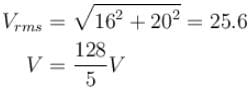The correct answer is: 128

*Answer can only contain numeric values
Alternating Current NAT Level - 2 - Question 2

### A LCR series circuit with 100Ω resistance is connected to an AC source of 200V and angular frequency 300 radians per second. When only the capacitance is removed, the current lags behind the voltage by 60°. When only the inductance is removed, the current leads the voltage by 60°. Calculate the average power dissipated in LCR circuit in watts.

Detailed Solution for Alternating Current NAT Level - 2 - Question 2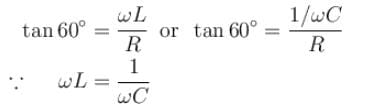Impedance of circuit = R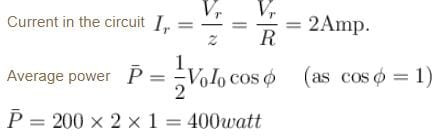The correct answer is: 200

*Answer can only contain numeric values
Alternating Current NAT Level - 2 - Question 3

### An inductor (XL = 2Ω) a capacitor (XC = 8Ω) and a resistance 8Ω are connected in series with an AC source. The voltage output of AC source is given by V = cos100πt,  then the impedance of the circuit (in Ω).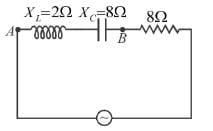Detailed Solution for Alternating Current NAT Level - 2 - Question 3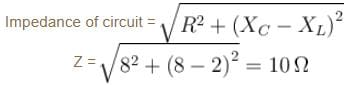The correct answer is: 10

*Answer can only contain numeric values
Alternating Current NAT Level - 2 - Question 4

LCR series, AC circuit operates at half its resonant frequency and at a power factor of 0.8. Then  the ratio of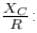Detailed Solution for Alternating Current NAT Level - 2 - Question 4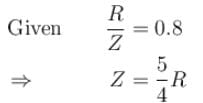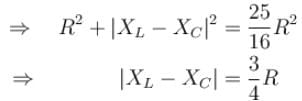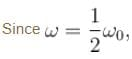resonant frequency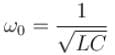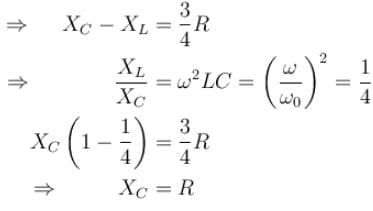The correct answer is: 1

*Answer can only contain numeric values
Alternating Current NAT Level - 2 - Question 5

In the LCR circuit shown if only L is removed, the current leads the supply voltage by 30°. If only C is removed the current lags the voltage by 60°. The resonant frequency is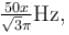then what is the value of x.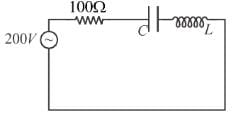Detailed Solution for Alternating Current NAT Level - 2 - Question 5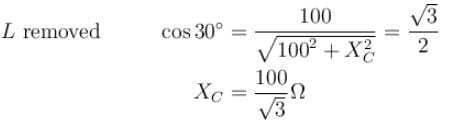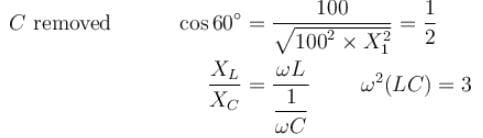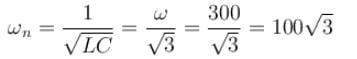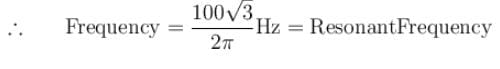The correct answer is: 3

*Answer can only contain numeric values
Alternating Current NAT Level - 2 - Question 6

The voltage time (V-t) graph for triangular wave having peak value V0 is as shown in figure. The rms value of V in time interval from t = 0  to T/4 is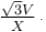Then, X is :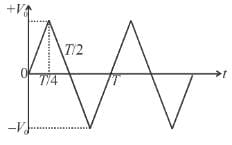Detailed Solution for Alternating Current NAT Level - 2 - Question 6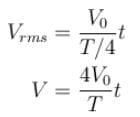⇒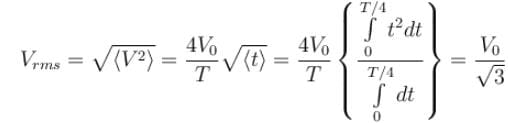The correct answer is: 3

*Answer can only contain numeric values
Alternating Current NAT Level - 2 - Question 7

The average value of current i = Im sin ωt from t = π/2ω to t = 3π/2ω is how many times of Im ?

Detailed Solution for Alternating Current NAT Level - 2 - Question 7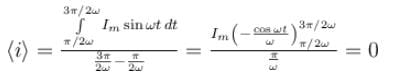The correct answer is: 0

*Answer can only contain numeric values
Alternating Current NAT Level - 2 - Question 8

In the circuit diagram shown, X= 100Ω, X= 200Ω and R = 100Ω. The effective current through the source is √N.  Then N is :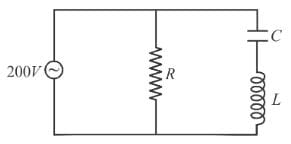Detailed Solution for Alternating Current NAT Level - 2 - Question 8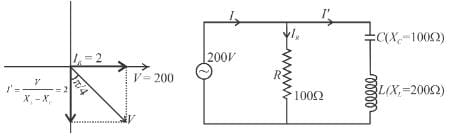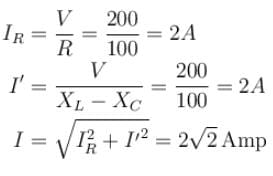The correct answer is: 8

*Answer can only contain numeric values
Alternating Current NAT Level - 2 - Question 9

Find the average value of current from t = 0 to t = 2π/ω, if the current varies as i = Imsinωt.

Detailed Solution for Alternating Current NAT Level - 2 - Question 9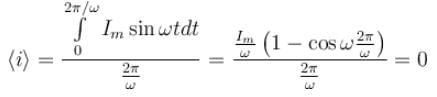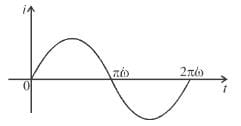It can be seen graphically that the area of i = t graph of one cycle is zero.
∴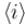is one cycle.
The correct answer is: 0

*Answer can only contain numeric values
Alternating Current NAT Level - 2 - Question 10

An inductor (XL = 2Ω), a capacitor (XC = 8Ω) and a resistance 8Ω are connected in series with an AC source. The instantaneous potential difference between A and B when it is half of the voltage output from source at that instant is x/5 volt, then x is.

Detailed Solution for Alternating Current NAT Level - 2 - Question 10

The current leads in phase by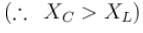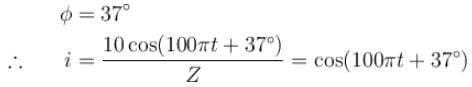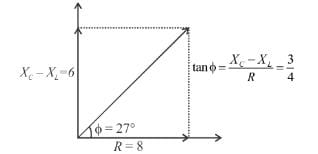The instantaneous potential difference across AB is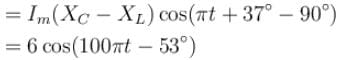The instantaneous potential difference across AB is half of source voltage.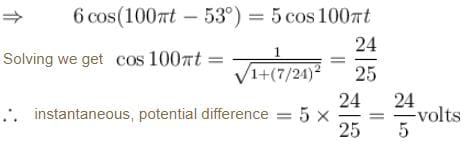The correct answer is: 24

## Topic wise Tests for IIT JAM Physics

217 tests
 Use Code STAYHOME200 and get INR 200 additional OFF Use Coupon Code
Information about Alternating Current NAT Level - 2 Page
In this test you can find the Exam questions for Alternating Current NAT Level - 2 solved & explained in the simplest way possible. Besides giving Questions and answers for Alternating Current NAT Level - 2, EduRev gives you an ample number of Online tests for practice

217 tests